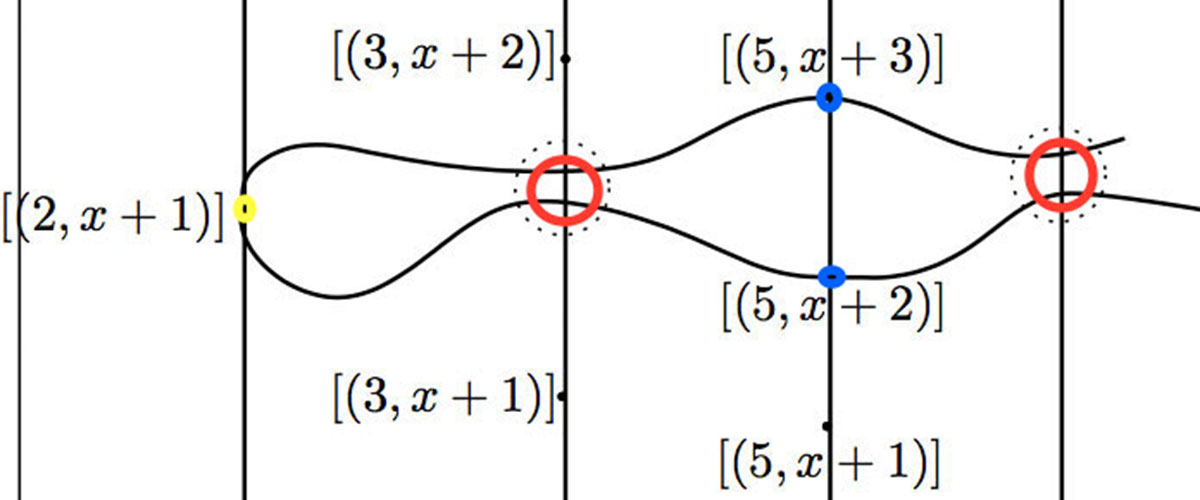Geometers will tell you there are two ways to introduce affine schemes.

You can use structure sheaves. That is, compute all prime ideals of your ring and turn them into a space. Then, put a sheaf of rings on this space by localisation. You’ll get your ring back taking global sections.

Or, you might try the ‘functor of points’. That is, you take any other ring. Compute all ring-morphisms from yours to that one. You’ll recover your ring from Yoneda’s lemma.

And here’s the funny part.

Scheme-theorists claim there’s no differences between these two approaches. They are ‘equivalent’, as they prefer to say.

Do you believe them?

Let’s look at an example.

Take the ring of all polynomials with integer coefficients, $\mathbb{Z}[x]$.

Do you know all its prime ideals?

Sure, you’ll say.

There’s zero because it’s a domain. Then there are the ‘curves’. These are all prime numbers and all irreducible polynomials because it’s a UFD.

And then there are the ‘points’. They depend on a prime number $p$ and an irreducible polynomial which does not factor over $p$.

Not exactly rocket science, is it?

Okay, now let’s take them all together into a space.

Can you picture the intersection points of different curves? Let’s keep it simple. Take the curve given by a prime number $p$ and the one given by an irreducible polynomial $F(x)$. How do they intersect?

Easy! They are the factors of $F(x)$ modulo $p$.Right, but can you picture this pattern for all prime numbers at once?

That depends on $F(x)$. David Mumford sketched the situation for $x^2+1$.

If $-1$ is a square modulo $p$, then $F(x)$ splits in two factors giving two points, such as along $5$. If not, $F(x)$ remains irreducible over $p$ and gives a thicker point like over $3$ or $7$. Except for the ‘odd’ case over $2$ where $F(x)$ is a square. Gauss knew already the situation for every prime.

That’s a lot more difficult. Chebotarev knew how to get their Galois group from the factors at all primes.

So, you’ll need to solve deep problems in number theory before you can picture this space. The structure of the absolute Galois group $Gal(\overline{\mathbb{Q}}/\mathbb{Q})$ to name one.

I’m afraid nobody understands the space of all prime ideals of $\mathbb{Z}[x]$ completely, let alone its structure sheaf.

What about the other approach? Let’s try to understand the functor of points of $\mathbb{Z}[x]$.

Take any ring $R$. We need to figure out all ring-maps $\mathbb{Z}[x] \rightarrow R$. But, we know such a map once we know the image of $x$. That is, there are as many ring-maps as there are elements in the set $R$.

Forgetting all about addition and multiplication on $R$. It is just the forgetful functor from rings to sets.

And they claim this is equivalent to solving deep problems in number theory?

Forgetting can’t be that hard, can it?

Published in geometry